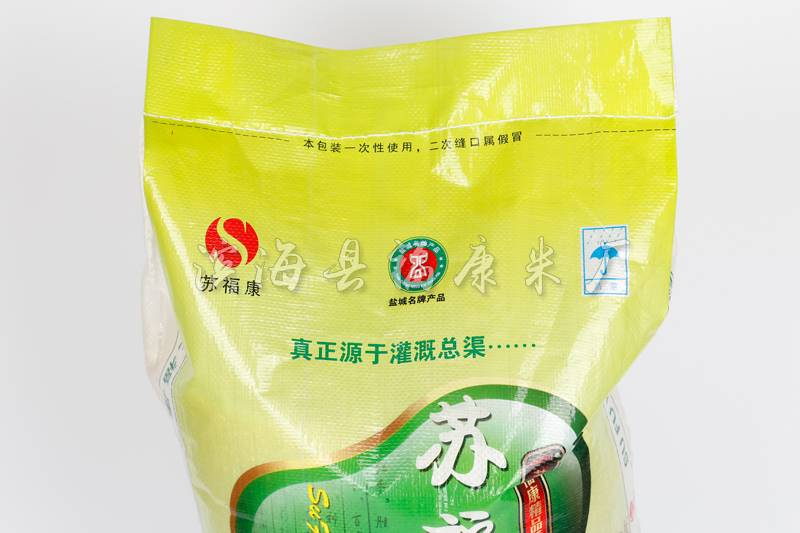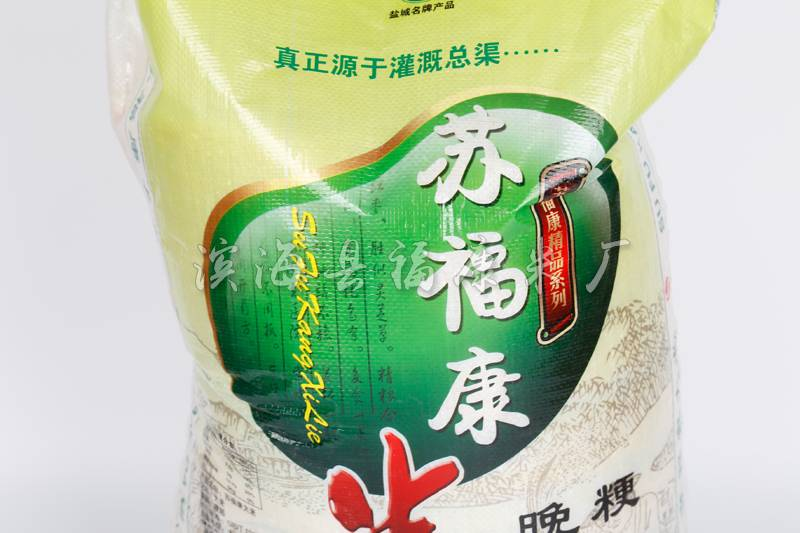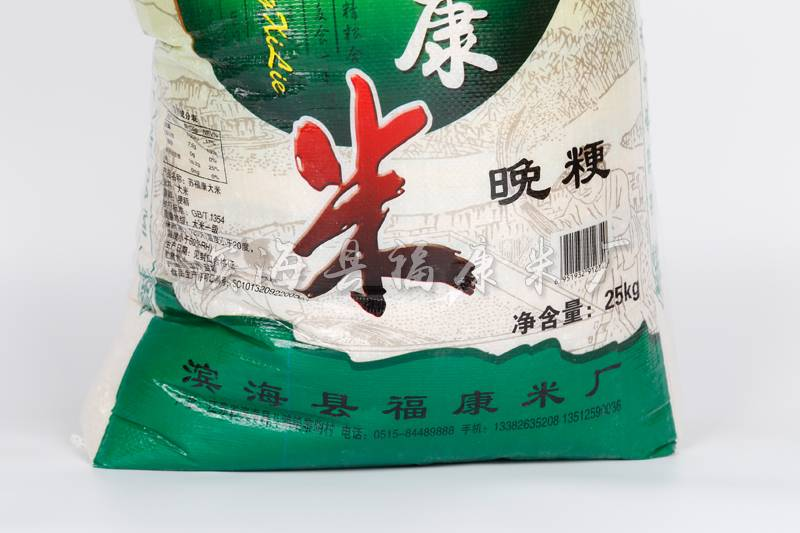#### 来自大自然的馈赠颗粒饱满 • 香甜美味• 海福康
• {dede:sql sql='Select * from dede_arctype where reid=~id~'}
[field:typename/]
{dede:sql sql='Select * from dede_arctype where reid=~id~'}
• 苏福康
• {dede:sql sql='Select * from dede_arctype where reid=~id~'}
[field:typename/]
{dede:sql sql='Select * from dede_arctype where reid=~id~'}
• 射阳大米
• {dede:sql sql='Select * from dede_arctype where reid=~id~'}
[field:typename/]
{dede:sql sql='Select * from dede_arctype where reid=~id~'}

# 苏福康晚粳米25kg

13512590036（孟宪光）
13912581989（张孝弘）
13382633258（孟庆文）
• 产品详情
• 联系我们颗粒饱满：产地直供，粒粒甄选   晶莹剔透：色泽明亮，质地通透   香气宜人：香味甘甜，蒸煮时有米香味   软糯醇香：自然稻谷的清香，软糯有弹性

• *联系人：
• *手机：
• 公司名称：
• 邮箱：
• 电话：
• 地址：
• *采购意向：